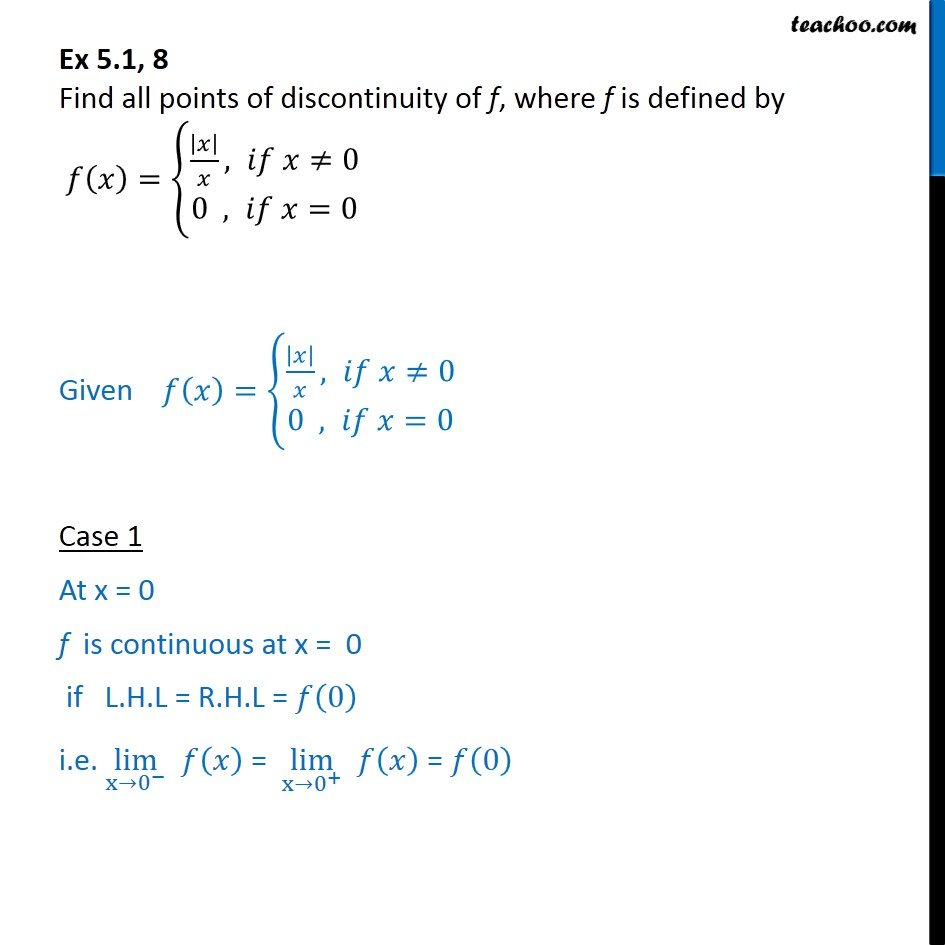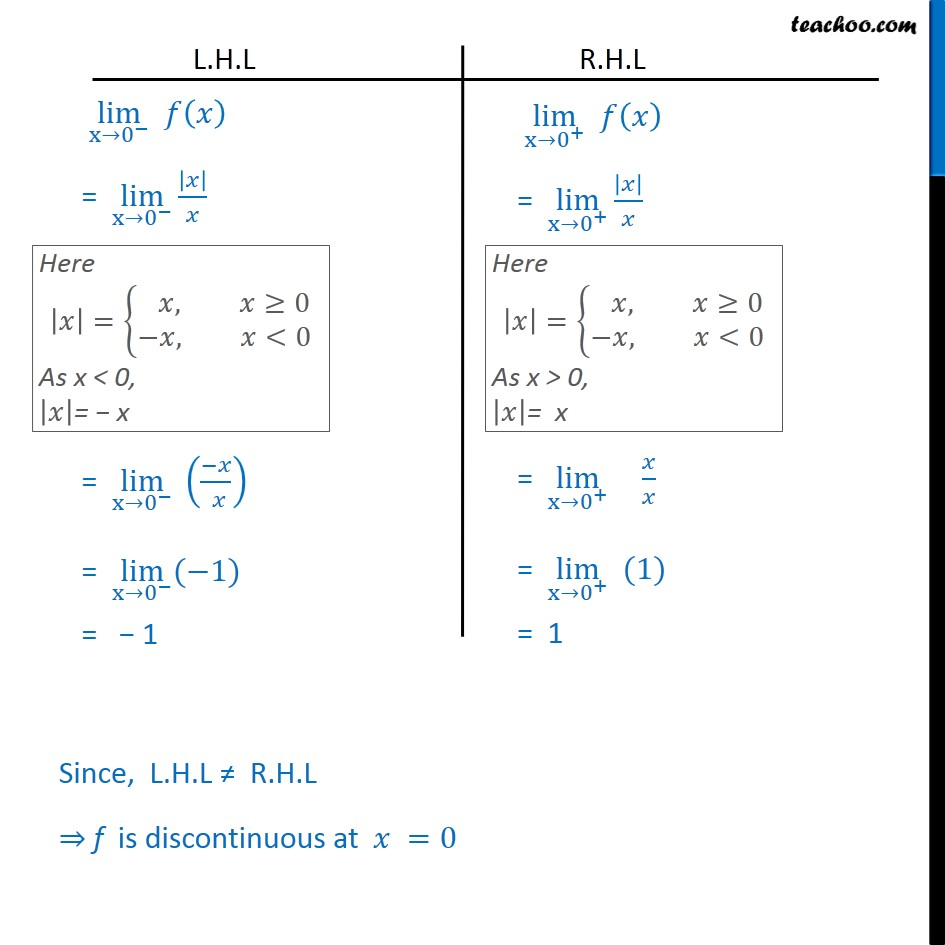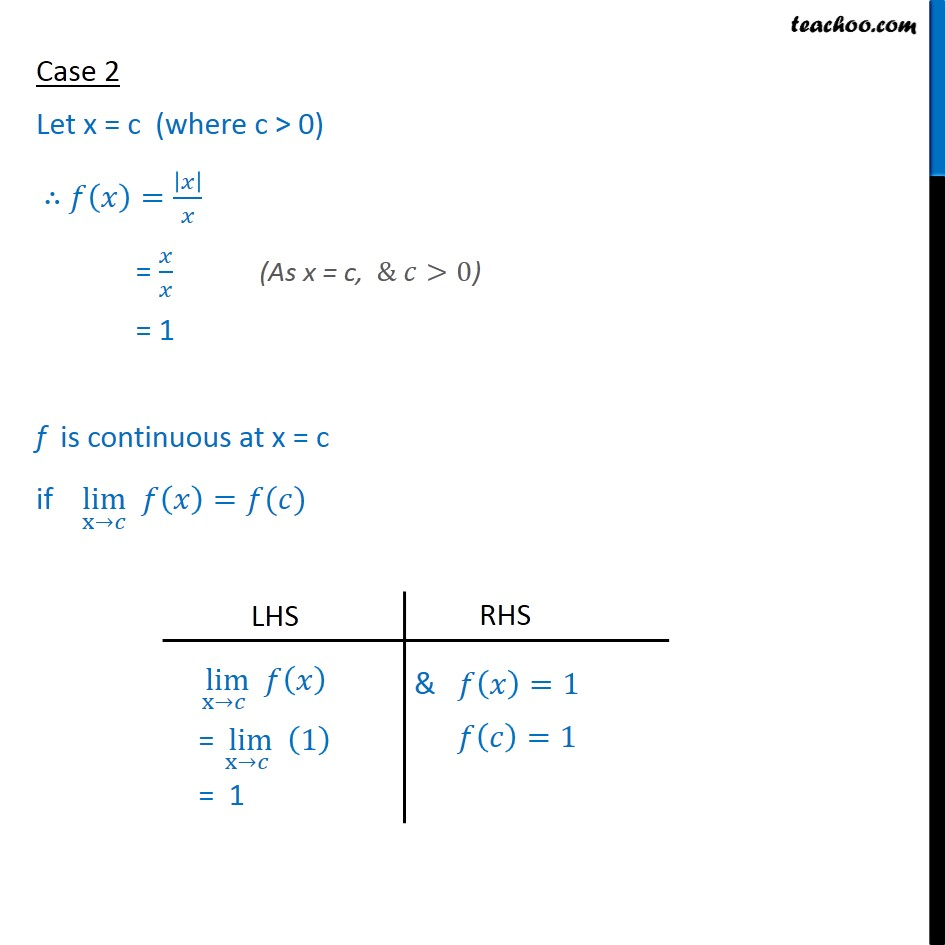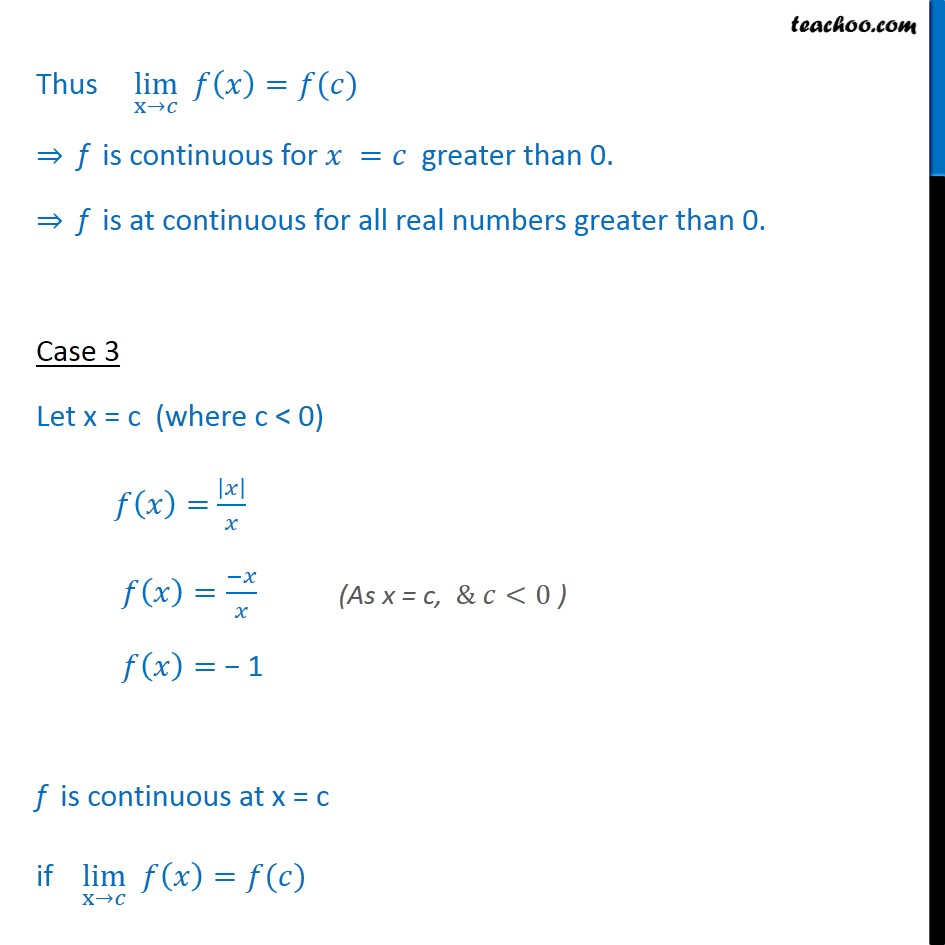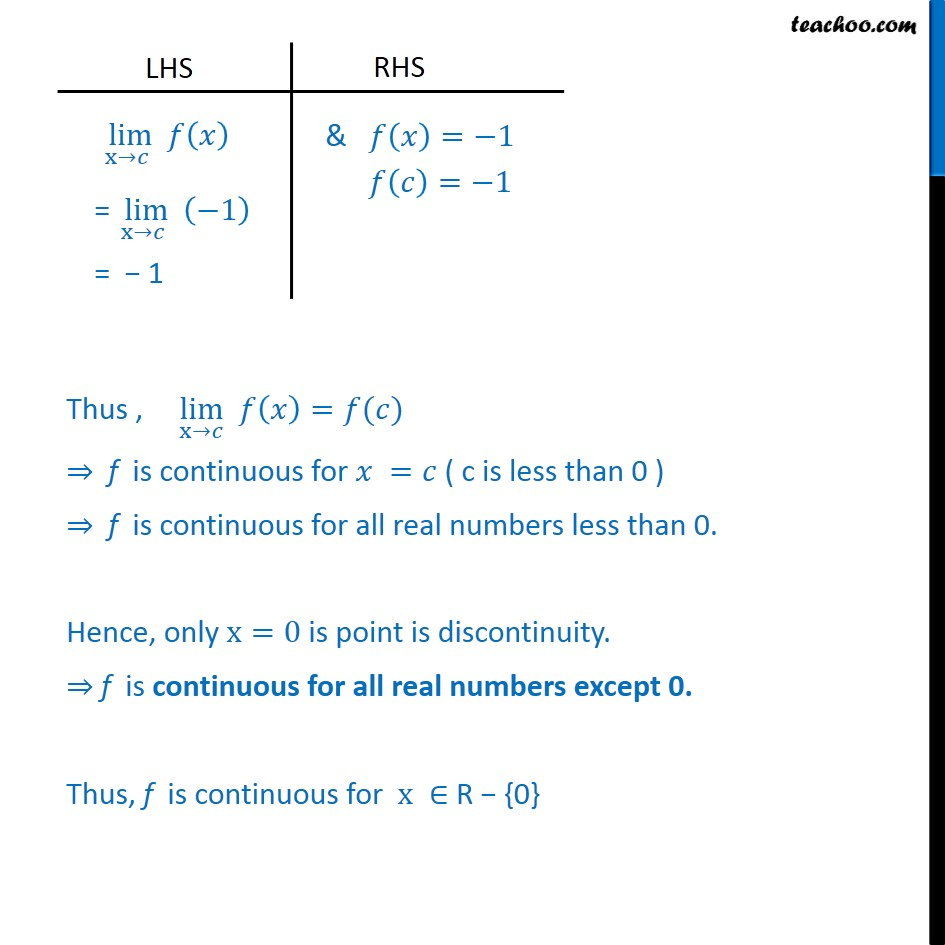1. Chapter 5 Class 12 Continuity and Differentiability
2. Serial order wise
3. Ex 5.1

Transcript

Ex 5.1, 8 Find all points of discontinuity of f, where f is defined by 𝑓﷐𝑥﷯=﷐﷐﷐﷐𝑥﷯﷮𝑥﷯, 𝑖𝑓 𝑥≠0﷮&0 , 𝑖𝑓 𝑥=0﷯﷯ Given 𝑓﷐𝑥﷯=﷐﷐﷐﷐𝑥﷯﷮𝑥﷯, 𝑖𝑓 𝑥≠0﷮&0 , 𝑖𝑓 𝑥=0﷯﷯ Case 1 At x = 0 f is continuous at x = 0 if L.H.L = R.H.L = 𝑓﷐0﷯ i.e. ﷐lim﷮x→﷐0﷮−﷯﷯ 𝑓﷐𝑥﷯ = ﷐lim﷮x→﷐0﷮+﷯﷯ 𝑓﷐𝑥﷯ = 𝑓﷐0﷯ Since, L.H.L ≠ R.H.L ⇒ f is discontinuous at 𝑥 =0 Case 2 Let x = c (where c > 0) ∴ 𝑓﷐𝑥﷯=﷐﷐𝑥﷯﷮𝑥﷯ = ﷐𝑥﷮𝑥﷯ = 1 f is continuous at x = c if ﷐lim﷮x→𝑐﷯ 𝑓﷐𝑥﷯=𝑓(𝑐) Thus ﷐lim﷮x→𝑐﷯ 𝑓﷐𝑥﷯=𝑓(𝑐) ⇒ f is continuous for 𝑥 =𝑐 greater than 0. ⇒ f is at continuous for all real numbers greater than 0. Case 3 Let x = c (where c < 0) 𝑓﷐𝑥﷯= ﷐﷐𝑥﷯﷮𝑥﷯ 𝑓﷐𝑥﷯=﷐−𝑥﷮𝑥﷯ 𝑓﷐𝑥﷯= − 1 f is continuous at x = c if ﷐lim﷮x→𝑐﷯ 𝑓﷐𝑥﷯=𝑓(𝑐) Thus , ﷐lim﷮x→𝑐﷯ 𝑓﷐𝑥﷯=𝑓(𝑐) ⇒ f is continuous for 𝑥 =𝑐 ( c is less than 0 ) ⇒ f is continuous for all real numbers less than 0. Hence, only x=0 is point is discontinuity. ⇒ f is continuous for all real numbers except 0. Thus, f is continuous for x ∈ R − {0}

Ex 5.1